×#### Thank you for registering.

One of our academic counsellors will contact you within 1 working day.

Click to Chat

1800-1023-196

+91-120-4616500

CART 0

• 0

MY CART (5)

Use Coupon: CART20 and get 20% off on all online Study Material

ITEM
DETAILS
MRP
DISCOUNT
FINAL PRICE
Total Price: Rs.

There are no items in this cart.
Continue Shopping• Complete JEE Main/Advanced Course and Test Series
• OFFERED PRICE: Rs. 15,900
• View Details

```Chapter 3: Pair of Linear Equations in Two Variables Exercise – 3.5

Question: 1

In each of the following systems of equation determine whether the system has a unique solution, no solution or infinite solutions. In case there is a unique solution,

x − 3y − 3 = 0,  x − 3y − 3 = 0

3x − 9y − 2 = 0, 3x − 9y − 2 = 0

Solution:

The given system may be written as

x − 3y − 3 = 0

3x − 9y − 2 = 0

The given system of equation is of the form

a2x + b2y − c2 = 0 a2x + b2y − c2 = 0

Where, a1 = 1, b1 = −3, c1 = −3

a2 = 3, b2 = −9, c2 = −2

We have,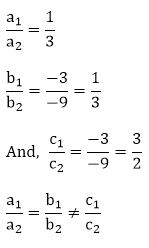Therefore, the given equation has no solution.

Question: 2

In each of the following systems of equation determine whether the system has a unique solution, no solution or infinite solutions. In case there is a unique solution,

2x + y − 5 = 0

4x + 2y − 10 = 0

Solution:

The given system may be written as

2x + y − 5 = 0 4x + 2y − 10 = 0

The given system of equation is of the form

a1x + b1y − c1 = 0 a2x + b2y − c2 = 0

Where, a1 = 2, b1 = 1, c1 = −5

a2 = 4, b2 = 2, c2 = −10

We have,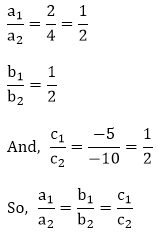Therefore, the given equation has infinitely many solution.

Question: 3

In each of the following systems of equation determine whether the system has a unique solution, no solution or infinite solutions. In case there is a unique solution,

3x − 5y = 20

6x − 10y = 40

Solution:

The given system may be written as

3x − 5y = 20 6x − 10y = 40

The given system of equation is of the form

a1x + b1y − c1 = 0 a2x + b2y − c2 = 0

Where, a1 = 3, b1 = −5, c1 = − 20

a2 = 6, b2 = −10, c2 = − 40

We have,Therefore, the given equation has infinitely many solution.

Question: 4

In each of the following systems of equation determine whether the system has a unique solution, no solution or infinite solutions. In case there is a unique solution,

x − 2y − 8 = 0

5x − 10y − 10 = 0

Solution:

The given system may be written as

x − 2y − 8 = 0 5x − 10y − 10 = 0

The given system of equation is of the form

a1x + b1y − c1 = 0 a2x + b2y − c2 = 0

Where, a1 = 1, b1 = −2, c1 = −8

a2 = 5, b2 = −10, c2 = −10

We have,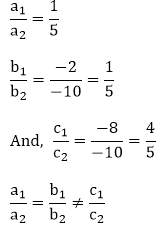Therefore, the given equation has no solution.

Question: 5

Find the value of k for each of the following system of equations which have a unique solution

kx + 2y − 5 = 0

3x + y − 1 = 0

Solution:

The given system may be written as

kx + 2y − 5 = 0

3x + y − 1 = 0

The given system of equation is of the form

a1x + b1y − c1 = 0

a2x + b2y − c2 = 0

Where, a1 = k, b1 = 2, c1 = −5

a2 = 3, b2 = 1, c2 = −1

For unique solution, we haveTherefore, the given system will have unique solution for all real values of k other than 6.

Question: 6

Find the value of k for each of the following system of equations which have a unique solution

4x + ky + 8 = 0

2x + 2y + 2 = 0

Solution:

The given system may be written as

4x + ky + 8 = 0 2x + 2y + 2 = 0

The given system of equation is of the form

a1x +b1y − c1 = 0 a2x + b2y − c2 = 0

Where, a1 = 4, b1 = k, c1 = 8

a2 = 2, b2 = 2, c2 = 2

For unique solution, we haveTherefore, the given system will have unique solution for all real values of k other than 4.

Question: 7

Find the value of k for each of the following system of equations which have a unique solution

4x − 5y = k

2x − 3y = 12

Solution:

The given system may be written as

4x − 5y − k = 0

2x − 3y − 12 = 0

The given system of equation is of the form

a1x + b1y − c1 = 0 a2x + b2y − c2 = 0

Where, a1 = 4, b1 = −5, c1 = −k

a2 = 2, b2 = -3, c2 = -12

For unique solution, we have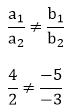⇒ k can have any real values.

Therefore, the given system will have unique solution for all real values of k.

Question: 8

Find the value of k for each of the following system of equations which have a unique solution

x + 2y = 3

5x + ky + 7 = 0

Solution:

The given system may be written as

x + 2y = 3

5x + ky + 7 = 0

The given system of equation is of the form

a1x + b1y − c1 = 0 a2x + b2y − c2 = 0

Where a1 = 1, b1 = 2, c1 = −3

a2 = 5, b2 = k, c2 = 7

For unique solution, we haveTherefore, the given system will have unique solution for all real values of k other than 10.

Question: 9

Find the value of k for which each of the following system of equations having infinitely many solution:

2x + 3y − 5 = 0

6x − ky − 15 = 0

Solution:

The given system may be written as

2x + 3y − 5 = 0 6x − ky − 15 = 0

The given system of equation is of the form

a1x + b1y − c1 = 0 a2x + b2y − c2 = 0

Where, a1 = 2, b1 = 3, c1 = −5

a2 = 6, b2 = k, c2 = −15

For unique solution, we have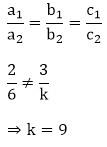Therefore, the given system of equation will have infinitely many solutions, if k = 9.

Question: 10

Find the value of k for which each of the following system of equations having infinitely many solution:

4x + 5y = 3

x + 15y = 9

Solution:

The given system may be written as

4x + 5y = 3

kx +15y = 9

The given system of equation is of the form

a1x + b1y − c1 = 0 a2x + b2y − c2 = 0

Where, a1 = 4, b1 = 5, c1 = 3

a2 = k, b2 = 15, c2 = 9

For unique solution, we have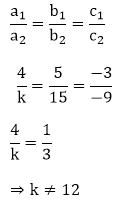Therefore, the given system will have infinitely many solutions if k = 12.

Question: 11

Find the value of k for which each of the following system of equations having infinitely many solution:

kx − 2y + 6 = 0

4x + 3y + 9 = 0

Solution:

The given system may be written as

kx − 2y + 6 = 0 4x + 3y + 9 = 0

The given system of equation is of the form

a1x + b1y − c1 = 0 a2x + b2y − c2 = 0

Where, a1 = k, b1 = −2, c1 = 6

a2 = 4, b2 = −3, c2 = 9

For unique solution, we have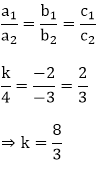Therefore, the given system of equations will have infinitely many solutions, if k = 8/3.

Question: 12

Find the value of k for which each of the following system of equations having infinitely many solution:

8x + 5y = 9

kx + 10y = 19

Solution:

The given system may be written as

8x + 5y = 9 kx + 10y = 19

The given system of equation is of the form

a1x + b1y − c1 = 0 a2x + b2y − c2 =0

Where, a1 = 8, b1 = 5, c1 = −9

a2 = k, b2 = 10, c2 = −18 a2 = k, b2 = 10,c2 = −18

For unique solution, we have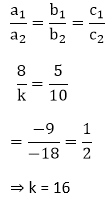Therefore, the given system of equations will have infinitely many solutions, if k = 16.

Question: 13

Find the value of k for which each of the following system of equations having infinitely many solution:

2x − 3y = 7

(k + 2)x − (2k + 1)y = 3(2k − 1)

Solution:

The given system may be written as

2x − 3y = 7 (k + 2)x − (2k + 1)y = 3(2k − 1)

The given system of equation is of the form

a1x + b1y − c1 = 0 a2x + b2y − c2 = 0

Where, a1 = 2, b1 = −3, c1 = −7

a2 = k, b2 = − (2k + 1), c2 = −3(2k − 1)

For unique solution, we have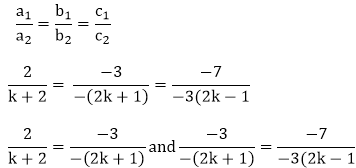⇒ 2(2k + 1) = 3(k + 2) and 3 × 3(2k − 1) = 7(2k + 1)

⇒ 4k + 2 = 3k + 6 and 18k − 9 = 14k + 7

⇒ k = 4 and 4k = 16

⇒ k = 4

Therefore, the given system of equations will have infinitely many solutions, if k = 4.

Question: 14

Find the value of k for which each of the following system of equations having infinitely many solution:

2x + 3y = 2

(k + 2)x + (2k + 1)y = 2(k − 1)

Solution:

The given system may be written as

2x + 3y = 2 (k + 2)x + (2k + 1)y = 2(k − 1)

The given system of equation is of the form

a1x + b1y − c1 = 0 a2x + b2y − c2 = 0

Where, a1 = 2, b1 = 3,c1 = −2

a2 = (k + 2), b2 = (2k + 1),c2 = −2(k − 1)

For unique solution, we have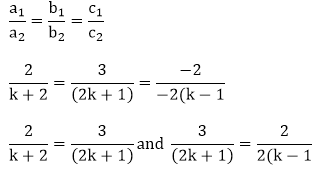⇒ 2(2k + 1) = 3(k + 2) and 3(k − 1) = (2k + 1)

⇒ 4k + 2 = 3k + 6 and 3k − 3 = 2k + 1

⇒ k = 4 and k = 4

Therefore, the given system of equations will have infinitely many solutions, if k = 4.

Question: 15

Find the value of k for which each of the following system of equations having infinitely many solution:

x + (k + 1)y = 4

(k + 1)x + 9y = (5k + 2)

Solution:

The given system may be written as

x + (k + 1)y = 4 (k + 1)x + 9y = (5k + 2)

The given system of equation is of the form

a1x + b1y − c1 = 0 a2x + b2y − c2 = 0

Where, a1 = 1, b1 = (k + 1), c1 = −4

a2 = (k + 1), b2 = 9, c2 = − (5k + 2)

For unique solution, we have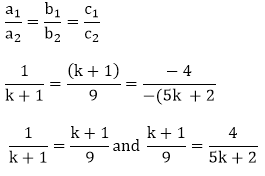⇒ 9 = (k + 1)2 and (k + 1)(5k + 2) = 36

⇒ 9 = k2 + 2k + 1 and 5k2 + 2k + 5k + 2 = 36

⇒ k2 + 2k − 8 = 0 and 5k2 + 7k − 34 = 0

⇒ k2 + 4k − 2k − 8 = 0 and 5k2 + 17k − 10k − 34 = 0

⇒ k(k + 4) −2 (k + 4) = 0 and (5k + 17) − 2 (5k + 17) = 0

⇒ (k + 4)(k − 2) = 0 and (5k + 17)(k − 2) = 0

⇒ k = - 4 or k = 2 and k = -17/5 or k = 2

Thus, k = 2 satisfies both the condition.

Therefore, the given system of equations will have infinitely many solutions, if k = 2.

Question: 16

Find the value of k for which each of the following system of equations having infinitely many solution:

kx + 3y = 2k + 1

2(k + 1)x + 9y = (7k + 1)

Solution:

The given system may be written as

kx + 3y = 2k + 1 2(k + 1)x + 9y = (7k + 1)

The given system of equation is of the form

a1x + b1y − c1 = 0 a2x + b2y − c2 = 0

Where, a1 = k, b1 = 3, c1 = −(2k + 1)

a2 = 2(k + 1), b2 = 9, c2 = −(7k + 1)

For unique solution, we have⇒ 9k = 3 × 2(k + 1) and 3(7k + 1) = 9(2k + 1)

⇒ 9k − 6k = 6 and 21k − 18k = 9 − 3

⇒ 3k = 6 ⇒ k = 2 and k = 2

Therefore, the given system of equations will have infinitely many solutions, if k = 2.

Question: 17

Find the value of k for which each of the following system of equations having infinitely many solution:

2x + (k − 2)y = k

6x + (2k − 1)y = (2k + 5)

Solution:

The given system may be written as

2x +( k − 2)y = k 6x + (2k − 1)y = (2k + 5)

The given system of equation is of the form

a1x + b1y − c1 = 0 a2x + b2y − c2 = 0

Where,  a1 = 2,b1 = (k − 2),c1 = −k

a2 = 6,b2 = (2k − 1),c2 = −(2k + 5)

For unique solution, we have⇒ 2k − 3k = −6 + 1 and k + k = 10

⇒ −k = −5 and 2k = 10 ⇒ k = 5 and k = 5

Therefore, the given system of equations will have infinitely many solutions, if k = 5.

Question: 18

Find the value of k for which each of the following system of equations having infinitely many solution:

2x + 3y = 72x + 3y = 7

(k + 1)x + (2k − 1)y = (4k + 1)

Solution:

The given system may be written as

2x + 3y = 7 (k + 1)x + (2k − 1)y = (4k + 1)

The given system of equation is of the form

a1x + b1y − c1 = 0 a2x + b2y − c2 = 0

Where,  a1 = 2, b1 = 3, c1 = −7

a2 = k + 1, b2 = 2k − 1, c2 = −(4k + 1)

For unique solution, we have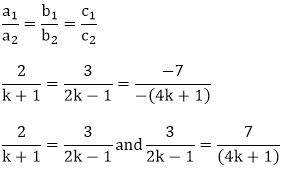Extra close brace or missing open brace

⇒ 4k − 2 = 3k + 3 and 12k + 3 = 14k − 7

⇒ k = 5 and 2k = 10 ⇒ k = 5 and k = 5

Therefore, the given system of equations will have infinitely many solutions, if k = 5.

Question: 19

Find the value of k for which each of the following system of equations having infinitely many solution:

2x + 3y = k

(k − 1)x + (k + 2)y = 3k

Solution:

The given system may be written as

2x + 3y = k (k − 1)x + (k + 2)y = 3k

The given system of equation is of the form

a1x + b1y − c1 = 0 a2x + b2y − c2 = 0

Where, a1 = 2,b1 = 3, c1 = −k

a2 = k − 1, b2 = k + 2, c2 = −3k

For unique solution, we have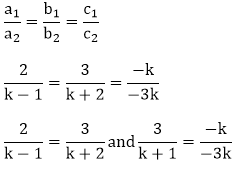Extra close brace or missing open brace

⇒ 2k + 4 = 3k − 3 and 9 = k + 2 ⇒ 2k + 4 = 3k − 3 and 9 = k + 2 ⇒ k = 7 and k = 7

Therefore, the given system of equations will have infinitely many solutions, if k = 7.

Question: 20

Find the value of k for which the following system of equation has no solution:

kx − 5y = 2

6x + 2y = 7

Solution:

The given system may be written as

kx − 5y = 2 6x + 2y = 7

The given system of equation is of the form

a1x + b1y − c1 = 0 a2x + b2y − c2 = 0

Where, a1 = k, b1 = −5, c1 = −2

a2 = 6 b2 = 2, c2 = −7

For no solution, we have⇒ 2k = -30 ⇒ k = -15

Therefore, the given system of equations will have no solutions, if k = −15.

Question: 21

Find the value of k for which the following system of equation has no solution:

x + 2y = 0

2x + ky = 5

Solution:

The given system may be written as

x2y = 0 2x + ky = 5

The given system of equation is of the form

a1x + b1y − c1 = 0 a2x + b2y − c2 = 0

Where, a1 = 1, b1 = 2, c1 = 0

a2 = 2, b2 = k, c2 = −5

For no solution, we have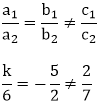⇒ k = 4

Therefore, the given system of equations will have no solutions, if k = 4.

Question: 22

Find the value of k for which the following system of equation has no solution:

3x − 4y + 7 = 0

kx + 3y − 5 = 0

Solution:

The given system may be written as

3x − 4y + 7 = 0 kx + 3y − 5 = 0

The given system of equation is of the form

a1x + b1y − c1 = 0 a2x + b2y − c2 = 0

Where, a1 = 3, b1 = −4, c1 = 7

a2 = k, b2 = 3, c2 = −5

For no solution, we haveTherefore, the given system of equations will have no solutions, if k = - 9 /4.

Question: 23

Find the value of k for which the following system of equation has no solution:

2x − ky + 3 = 0

3x + 2y − 1 = 0

Solution:

The given system may be written as

2x − ky + 3 = 0 3x + 2y − 1 = 0

The given system of equation is of the form

a1x + b1y − c1 = 0 a2x + b2y − c2 = 0

Where, a1 = 2, b1 = −k, c1 = 3

a2 = 3, b2 = 2, c2 = −1

For no solution, we have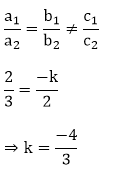Therefore, the given system of equations will have no solutions, if k = – 4/3.

Question: 24

Find the value of k for which the following system of equation has no solution:

2x + ky − 11 = 0

5x − 7y − 5 = 0

Solution:

The given system may be written as

2x + ky − 11 = 0 5x − 7y − 5 = 0

The given system of equation is of the form

a1x + b1y − c1 = 0 a2x + b2y − c2 = 0

Where, a1 = 2, b1 = k, c1 = −11

a2 = 5, b2 = −7, c2 = −5a2 = 5, b2 = −7, c2 = − 5

For no solution, we have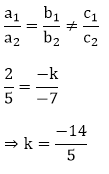Therefore, the given system of equations will have no solutions, if k = -14/5.

Question: 25

Find the value of k for which the following system of equation has no solution:

kx + 3y = 3

12x + ky = 6

Solution:

The given system may be written as

kx + 3y = 3 12x + ky = 6

The given system of equation is of the form

a1x + b1y − c1 = 0 a2x + b2y − c2 = 0

Where, a1 = k, b1 = 3, c1 = −3

a2 = 12, b2 = k, c2 = − 6

For no solution, we have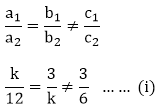⇒ k2 = 36 ⇒ k = + 6 or −6

From (i)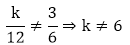Therefore, the given system of equations will have no solutions, if k = − 6.

Question: 26

For what value of a, the following system of equation will be inconsistent?

4x + 6y − 11 = 0

2x + ay − 7 = 0

Solution:

The given system may be written as

4x + 6y − 11 = 0 2x + ay − 7 = 0

The given system of equation is of the form

a1x + b1y − c1 = 0 a2x + b2y − c2 = 0

Where, a1 = 4, b1 = 6, c1 = −11

a2 = 2, b2 = a, c2 = −7

For unique solution, we have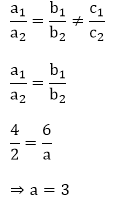Therefore, the given system of equations will be inconsistent, if a = 3.

Question: 27

For what value of a, the following system of equation have no solution?

ax + 3y = a − 3

12x + ay = a

Solution:

The given system may be written as

ax + 3y = a − 3 12x + ay = a

The given system of equation is of the form

a1x + b1y − c1 = 0 a2x + b2y − c2 = 0

Where, a1 = a, b1 = 3, c1 = - (a − 3)

a2 = 12, b2 = a, c2 = − a

For unique solution, we have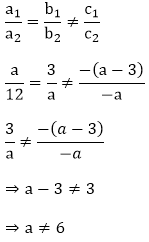And,⇒ a2 = 36

⇒ a = + 6 or – 6?

a  ≠ 6 ⇒ a = – 6

Therefore, the given system of equations will have no solution, if a = − 6.

Question: 28

Find the value of a, for which the following system of equation have

(i) Unique solution

(ii) No solution

kx + 2y = 5

3x + y = 1

Solution:

The given system may be written as

kx + 2y − 5 = 0 3x + y − 1 = 0

The given system of equation is of the form

a1x + b1y − c1 = 0 a2x + b2y − c2 = 0

Where, a1 = k, b1 = 2, c1 = −5

a2 = 3, b2 = 1, c2 = −1

(i) For unique solution, we have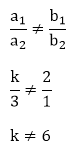Therefore, the given system of equations will have unique solution, if k ≠ 6 k ≠ 6.

(ii) For no solution, we have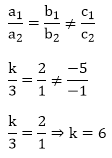Therefore, the given system of equations will have no solution, if a = 6.

Question: 29

For what value of c, the following system of equation have infinitely many solution (where c ≠ 0 c ≠ 0)?

6x + 3y = c − 3

12x + cy = c

Solution:

The given system may be written as

6x + 3y − (c − 3) = 0 12x + cy − c = 0

The given system of equation is of the form

a1x + b1y − c1 = 0 a2x + b2y − c2 = 0

Where, a1 = 6, b1 = 3, c1 = −(c − 3)

a2 = 12, b2 = c, c2 = - c

For infinitely many solution, we have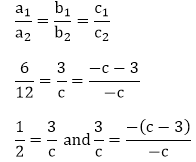⇒ c = 6 and c – 3 = 3

⇒ c = 6 and c = 6

Therefore, the given system of equations will have infinitely many solution, if c = 6.

Question: 30

Find the value of k, for which the following system of equation have

(i) Unique solution

(ii) No solution

(iii) Infinitely many solution

2x + ky = 1

3x − 5y = 7

Solution:

The given system may be written as

2x + ky = 1 3x − 5y = 7

The given system of equation is of the form

a1x + b1y − c1 = 0 a2x + b2y − c2 = 0

Where, a1 = 2, b1 = k, c1 = −1a1 = 2, b1 = k, c1 = −1

a2 = 3, b2 = −5, c2 = −7

(i) For unique solution, we haveTherefore, the given system of equations will have unique solution, if k ≠ -10/3.

(ii) For no solution, we haveTherefore, the given system of equations will have no solution, if k = -10)/3.

(iii) For the given system to have infinitely many solution, we haveSo there is no value of k for which the given system of equation has infinitely many solution.

Question: 31

For what value of k, the following system of equation will represent the coincident lines?

x + 2y + 7 = 0

2x + ky + 14 = 0

Solution:

The given system may be written as

x + 2y + 7 = 0 2x + ky + 14 = 0

The given system of equation is of the form

a1x + b1y − c1 = 0 a2x + b2y − c2 = 0

Where, a1 = 1, b1 = 2, c1 = 7

a2 = 2, b2 = k, c2 = 14

The given system of equation will represent the coincident lines if they have infinitely many solution.Therefore, the given system of equations will have infinitely many solution, if k = 4.

Question: 32

Find the value of k, for which the following system of equation have unique solution.

ax + by = c

lx + my = n

Solution:

The given system may be written as

ax + by − c = 0 lx + my − n = 0

The given system of equation is of the form

a1x + b1y − c1 = 0 a2x + b2y − c2 = 0

Where, a1 = a, b1 = b, c1 = − c

a2 = l, b2 = m, c2 = − n

For unique solution, we have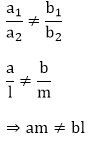Therefore, the given system of equations will have unique solution, if am ≠ bl.

Question: 33

Find the value of a and b such that the following system of linear equation have infinitely many solution:

(2a − 1)x + 3y − 5 = 0

3x + (b − 1)y − 2 = 0

Solution:

The given system of equation may be written as,

(2a − 1)x + 3y − 5 = 0 3x + (b − 1)y − 2 = 0

The given system of equation is of the form

a1x + b1y − c1 = 0 a2x + b2y − c2 = 0

Where, a1 = (2a − 1), b1 = 3, c1 = −5

a2 = 3, b2 = b − 1, c2 = −2

The given system of equation will have infinitely many solution, if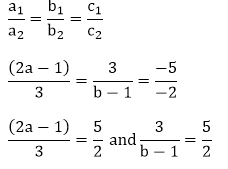⇒ 2(2a − 1) = 15 and 6 = 5(b − 1)

⇒ 4a − 2 = 15 and 6 = 5b − 5 ⇒ 4a = 17 and 5b = 11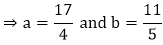Question: 34

Find the value of a and b such that the following system of linear equation have infinitely many solution:

2x − 3y = 7

(a + b)x − (a + b − 3)y = 4a + b

Solution:

The given system of equation may be written as,

2x − 3y − 7 = 0 (a + b)x − (a + b − 3)y − (4a + b) = 0

The given system of equation is of the form

a1x + b1y − c1 = 0 a2x + b2y − c2 = 0

Where, a1 = 2, b1 = −3, c1 = −7

a2 = (a + b), b2 = −(a + b − 3), c2 = −(4a + b)

The given system of equation will have infinitely many solution, if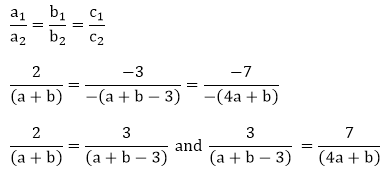⇒ 2(a + b − 3) = 3(a + b) and 3(4a + b) = 7(a + b − 3)

⇒ 2a + 2b − 6 = 3a + 3b and 12a + 3b = 7a + 7b − 21

⇒ a + b = −6 and 5a − 4b = −21

⇒ a = − 6 − b

Substituting the value of a in 5a − 4b = −21 we have

5( –  b – 6) – 4b = – 21

⇒ − 5b − 30 − 4b = − 21

⇒ 9b = − 9 ⇒ b = −1

As a = – 6 – b

⇒ a = − 6 + 1 = − 5

Hence the given system of equation will have infinitely many solution if

a = – 5 and b = –1.

Question: 35

Find the value of p and q such that the following system of linear equation have infinitely many solution:

2x − 3y = 9

(p + q)x + (2p − q)y = 3(p + q + 1)

Solution:

The given system of equation may be written as,

2x − 3y − 9 = 0 (p + q)x + (2p − q)y − 3(p + q + 1) = 0

The given system of equation is of the form

a1x + b1y − c1 = 0 a2x + b2y − c2 = 0

Where, a1 =2, b1 = 3, c1 = −9

a2 = (p + q), b2 = (2p − q), c2 = -3(p + q + 1)

The given system of equation will have infinitely many solution, if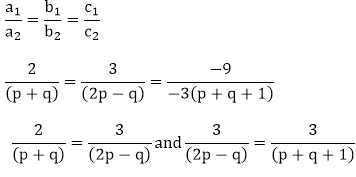2(2p - q) = 3(p + q) and (p + q + 1) = 2p - q

⇒ 4p - 2q = 3p + 3q and -p + 2q = -1

⇒ p = 5q and p - 2q = 1

Substituting the value of p in p - 2q = 1, we have

3q = 1

⇒ q = 1/3

Substituting the value of p in p = 5q we have

p = 5/3

Hence the given system of equation will have infinitely many solution if

p = 5/3 and q = 1/3.

Question: 36

Find the values of a and b for which the following system of equation has infinitely many solution:

(i)  (2a − 1)x + 3y = 5

3x + (b − 2)y = 3

(ii)  2x − (2a + 5)y = 5

(2b + 1)x − 9y = 15

(iii) (a − 1)x + 3y = 2

6x + (1 − 2b)y = 6

(iv) 3x + 4y = 12

(a + b)x + 2(a − b)y = 5a – 1

(v) 2x + 3y = 7

(a − 1)x + (a + 1)y = 3a − 1

(vi)  2x + 3y = 7

(a − 1)x + (a + 2)y = 3a

Solution:

(i) The given system of equation may be written as,

(2a − 1)x + 3y − 5 = 0 3x + (b − 2)y − 3 = 0

The given system of equation is of the form

a1x + b1y − c1 = 0 a2x + b2y − c2 = 0

Where, a1 = 2a − 1, b1 = 3, c1 = −5

a2 = 3, b2 = b − 2, c2 = -3(p + q + 1)

The given system of equation will have infinitely many solution, if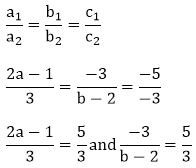2a - 1 = 5 and - 9 = 5(b - 2)

⇒ a = 3 and -9 = 5b - 10

a = 3 and b = 1/5

Hence the given system of equation will have infinitely many solution if

a = 3 and b = 1/5.

(ii)  The given system of equation may be written as,

2x − (2a + 5)y = 5 (2b + 1)x − 9y = 15

The given system of equation is of the form

a1x + b1y − c1 = 0 a2x + b2y − c2 = 0

Where, a1 = 2, b1 = - (2a + 5), c1 = −5

a2 = (2b + 1), b2 = −9, c2 = −15

The given system of equation will have infinitely many solution, ifHence the given system of equation will have infinitely many solution if

a = - 1 and b = 5/2.

(iii) The given system of equation may be written as,

(a − 1)x + 3y = 2 6x + (1 − 2b)y = 6

The given system of equation is of the form

a1x + b1y − c1 = 0 a2x + b2y − c2 = 0

Where, a1 = a-1, b1 = 3, c1 = −2

a2 = 6, b2 = 1 − 2b, c2 = −6

The given system of equation will have infinitely many solution, if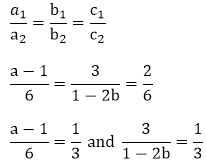⇒ a – 1 = 2 and 1 – 2b = 9

⇒ a - 1 = 2 and 1 - 2b = 9

⇒ a = 3 and b = -4

⇒ a = 3 and b = -4

Hence the given system of equation will have infinitely many solution if

a = 3 and b = −4.

(iv) The given system of equation may be written as,

3x + 4y − 12 = 0 (a + b)x + 2(a − b)y − (5a − 1) = 0

The given system of equation is of the form

a1x + b1y − c1 = 0 a2x + b2y − c2 = 0

Where, a1 = 3, b1 = 4, c1 = −12

a2 = (a + b), b2 = 2(a − b), c2 = – (5a − 1)

The given system of equation will have infinitely many solution, if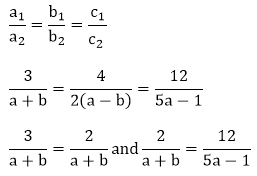⇒ 3(a - b) = 2a + 2b and 2(5a - 1) = 12(a - b)

⇒ a = 5b and -2a = -12b + 2

Substituting a = 5b in -2a = -12b + 2, we have

-2(5b) = -12b + 2

⇒ −10b = −12b + 2 ⇒ b = 1

Thus a = 5

Hence the given system of equation will have infinitely many solution if

a = 5 and b = 1.

(v) The given system of equation may be written as,

2x + 3y − 7 = 0 (a − 1)x + (a + 1)y − (3a − 1) = 0

The given system of equation is of the form

a1x + b1y − c1 = 0 a2x + b2y − c2 = 0

Where, a1 = 2, b1 = 3, c1 = −7

a2 = (a − 1), b2 = (a + 1), c2 = - (3a − 1)

The given system of equation will have infinitely many solution, if⇒ 2(a + 1) = 3(a - 1) and 3(3a - 1) = 7(a + 1)

⇒ 2a - 3a = -3 - 2 and 9a - 3 = 7a + 7

⇒ a = 5 and a = 5

Hence the given system of equation will have infinitely many solution if

a = 5 and b = 1.

(vi) The given system of equation may be written as,

2x + 3y − 7 = 0 (a − 1)x + (a + 2)y − 3a = 0

The given system of equation is of the form

a1x + b1y − c1 = 0 a2x + b2y − c2 = 0

Where, a1 = 2, b1 = 3, c1 = −7

a2 = (a − 1), b2 = (a + 2), c2 = −3a

The given system of equation will have infinitely many solution, if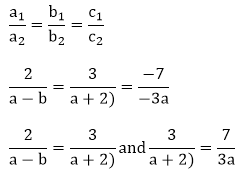⇒ 2(a + 2) = 3(a - 1) and 3(3a) = 7(a + 2)

⇒ 2a + 4 = 3a - 3 and 9a = 7a + 14

⇒ a = 7 and a = 7

Hence the given system of equation will have infinitely many solution if

a = 7and b = 1.
```### Course Features

• 728 Video Lectures
• Revision Notes
• Previous Year Papers
• Mind Map
• Study Planner
• NCERT Solutions
• Discussion Forum
• Test paper with Video Solution